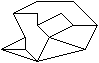Home Math Tim's Skiing SitesSelect an arbitrary number from 3 to 50 and draw this number of dots on a paper. Connect pairs of these dots by lines so that the lines do not cross and there are at least two lines leading to every dot. Now you have a polygon divided into smaller regions each of which is a triangle, quadrilateral, pentagon, or any other polygon. The dots become vertexes, and the lines become sides of these polygons.

Calculate the total number of sides, i.e. the total number of lines connecting two dots and not having other dots in between. Calculate the number of regions not overlapping each other. For instance, this octagon which is built up from 14 dots has 20 sides and 7 regions.Type these values in the text boxes below and press the Submit button. Wizard will discover what number you selected with 100% accuracy. Of course, you have to count the numbers correctly first.

 Total number of sides Number of regions